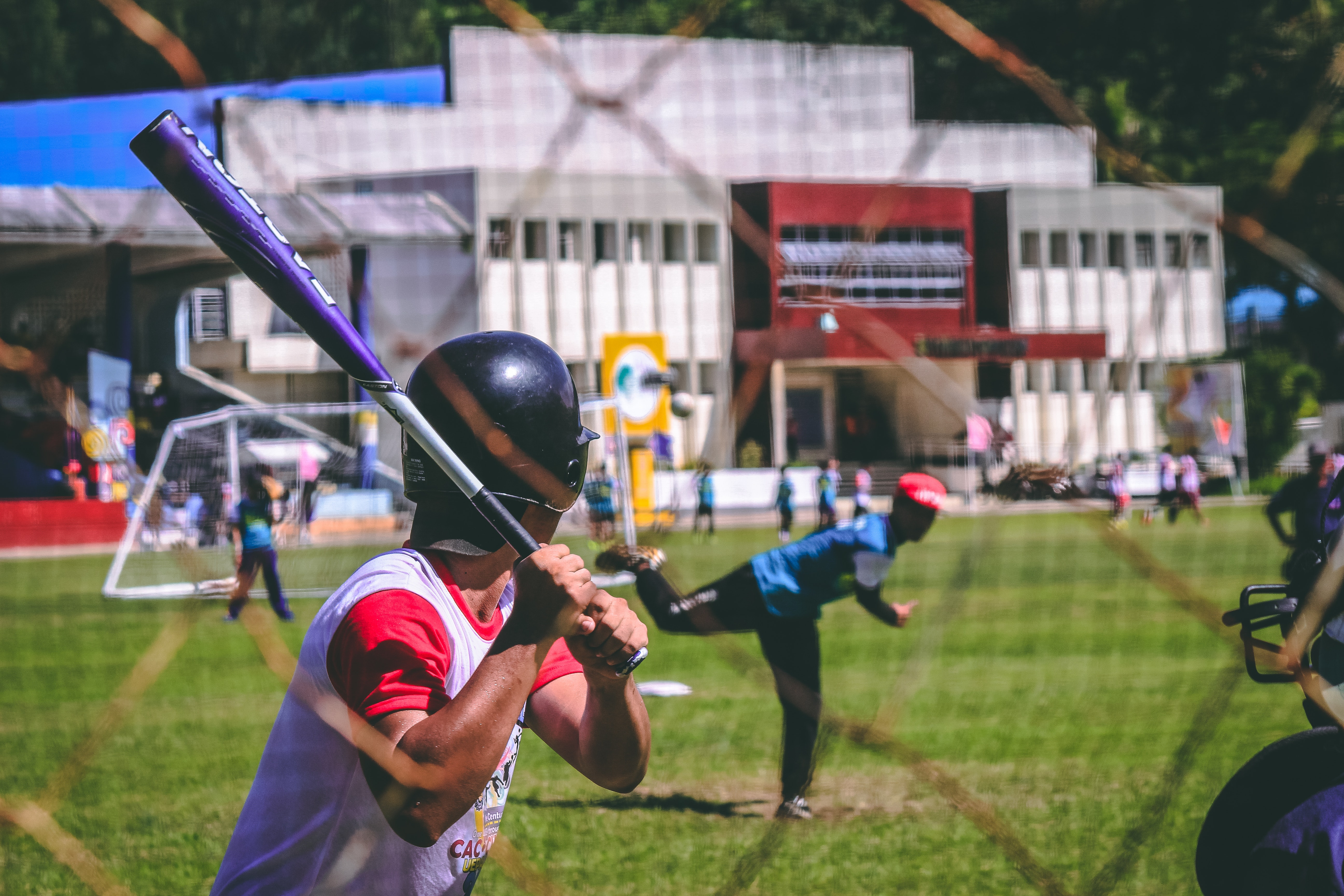# Impulse – definition, formula, unit

Last updated on April 16th, 2021 at 10:16 am

## Impulse Definition

If a force acts on a body for a very brief time then we say that an impulse is generated. As example, when we hit a ball with a bat for a brief period of time then an impulse is generated. Here a considerable amount of force acts on a body for a relatively shorter period of time. It is sometimes denoted with J .

## Impulse – understanding it

If the momentum of an object changes, then we can say that either the mass or the velocity or both change.

If the mass remains CONSTANT, as is most often the case, then the velocity changes and as a result acceleration occurs.

So, what produces acceleration? We know the answer is force.

[ Force F on an object = m x a = mass of the object x acceleration of the objecte. And acceleration a = rate of change of velocity = change of velocity per unit time]

The greater the net force acting on an object, the greater its change in velocity in a given time interval and, hence, the greater its change in momentum.

But something else is important in changing momentum: it is time—how long a time the force acts.

If we apply a brief force(force applied for a short duration of time) to a stalled vehicle, we produce a change in its momentum. Again if we apply the same force over an extended period of time, then we produce a greater change in the vehicle’s momentum.

A force sustained for a long time produces more change in momentum than does the same force applied briefly. So, both force and time interval are important in changing momentum.
The quantity force x time interval is called impulse. In shorthand notation, Impulse = J = F Δt
J changes momentum.

## Impulse formula

Impulse (J) = F .Δt

Here a force F acts on a body for a very small period of time Δt .

## SI unit – CGS unit

SI unit is Newton second

CGS unit is Dyne second

## Note:

Impulse( J ) is not something an object has, like momentum. It is what an object can provide or what it can experience when it interacts with some other object. An object cannot possess J just as it cannot possess force

Scroll to top
error: physicsTeacher.in